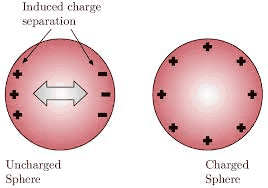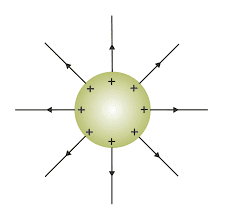# Surface charge of neutral solids

• I
• Philip Koeck

#### Philip Koeck

TL;DR Summary
Do neutral solids have a negative surface charge and does this distribute itself in a way so that the electrostatic field outside is zero everywhere no matter what shape the solid is?
Also referring to the post by @Kostik plus answers, I'm wondering about surface charges of neutral solids such as metals or carbon, for example.

I only want to discuss large scale effects so that the solid can be treated as continuous. The atomic structure is averaged out.
I also assume that this solid is not influenced by anything else. Let's say it's alone in empty space far from any other object.

It seems that every solid has to have a negative charge layer at the surface and the corresponding excess of positive charge below the surface.
At least this is the only way I can make sense of the mean inner potential (always positive) of a solid.

Assuming this is correct the field outside a sphere would still be zero due to Gauss' law and symmetry.
So would the field outside an infinitely large, plane surface.

But what about a more general solid object, for example a cylinder or a cone (or a potato-shaped object)?
Would the charges distribute themselves in such a way that the field is zero everywhere outside the object or would only the flux through a surface surrounding this object be zero?

Last edited:•Philip Koeck
View attachment 324582

Not really. In one of the examples above the solid is not neutral and the field outside isn't zero in either case.
I'm talking about a negative surface layer which is compensated by a positive layer further inside the solid.
For a sphere this gives a simple result (due to the symmetry): The field outside and inside is zero and the potential inside is higher than outside. The potential inside is known as the mean inner potential, which is at least numerically the same as the work function.

My question is whether the same works for all shapes (even without any symmetry).
Will the field outside always be zero and will the potential sufficiently far inside be constant?

And I'm only thinking of large objects (mm range) and averages over time. So I'm not considering charge oscillations.

I am not an expert on this, but my supposition is that the negative bias on the surface of a conductor is the Schottky Effect (https://en.wikipedia.org/wiki/Schottky_effect). At a small distance we will see the field arising from the negative charge, whilst at long distances, by superposition, we will see the fields of both negative and positive charges in the material, which add up to zero.
It is interesting that although a piece of metal appears from a distance to be uncharged, we are actually seeing very intense fields from the massive electron charge and an equal and opposite ionic charge. If now an accelerating field, such as from a battery, is placed transversly across the metal, the electrons immediately accelerate but the ions do not. The electrons now develop a field opposing the acceleration, which we see a small time later as radiation. In other words the fine balance of the very large superimposed fields has been broken.

•Philip Koeck